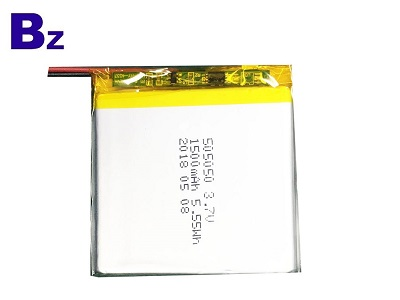# How to calculate the lithium content of lithium batteries?

According to the relevant provisions of the "Lithium Battery Air Transport Code" issued by the Civil Aviation Administration:1. When the battery in the spare battery or equipment is transported as baggage, the lithium content of the lithium metal or lithium alloy battery does not exceed 2g;

2. The rated energy of the lithium ion battery should not exceed 100Wh;

3. For large-scale lithium-ion batteries with rated energy greater than 100Wh and less than 160Wh, they can be carried with them after approval by the air operator, but the number of carriers must not exceed two.

4. The equipment containing lithium battery can be placed in checked baggage or hand luggage, and the spare lithium battery can only be placed in hand luggage.

Calculation method:

## 1, the rated energy calculation method:

A. If the nominal voltage (V) and nominal capacity (Ah) of the battery are known, the value of the rated energy can be calculated by calculation:

Wh = V x Ah

The nominal voltage and nominal capacity are usually marked on the battery.

B. If the battery is only marked with milliampere (mAh), divide this value by 1000 to get ampere-hours (Ah).

For example, the battery has a nominal voltage of 3.7V and a nominal capacity of 760mAh. Its rated energy is:

760mAh÷1000=0.76Ah

3.7V×0.76Ah=2.9Wh

## 2. Calculation method of lithium content in lithium battery:

If the battery is marked with current (mAh), the data can be divided by 1000 and multiplied by 0.3 to obtain the grams of lithium content of the battery.

For example, if the battery current is 4600mAh, the lithium content is 4600mAh ÷1000X0.3=1.38g, which is less than 2g, which meets safety standards.

Friend Links/ 百盈能源 / Bzbattery / Benzo Battery / Battery manufactory /